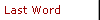Six Sigma and BeyondThomas PyzdekThe 1.5 Sigma ShiftInternal performance metrics often underestimate customer problems.

Few Six Sigma concepts create more confusion (or wrath) than the so-called 1.5 sigma shift. Over the years, I've come to discover that the majority of those writing about the subject really don't understand it. In this column, I'll try to set the record straight by explaining the true rationale behind this infamous "statistical correction."

The Standard Normal Distribution CurveThe standard normal distribution provides the starting point for discussion. This distribution has been extensively studied and tabularized, and many natural systems can be approximated with it. Although the distribution extends to infinity in both directions, drawings of the distribution usually only show the area from -3 standard deviations to +3 standard deviations because this range includes 99.73 percent of the data. Until Six Sigma became popular, all quality calculations were based on this distribution without any "adjustments." For example, assume surveys show that customers are unhappy when hold times exceed 15 minutes and that actual hold times are perfectly normally distributed, averaging 12 minutes with a standard deviation of one minute. The 15 minute specification is 3 standard deviations above the mean. Looking up the area of a normal distribution beyond +3 standard deviations, we predict that 0.135 percent of customer hold times will exceed the specification.

Six Sigma modifies this procedure by "adjusting" the calculated mean by 1.5 sigma before estimating the percentage out of specification. For the hold-time example, we would perform the calculations with an average hold time of 13.5 minutes instead of the calculated value of 12 minutes. This would give an estimate of 6.68 percent exceeding the specification.

There is a saying among engineers and scientists: All models are wrong, but some models are useful. The traditional normal model, for example, is certainly wrong, but it's often still useful. The question, then, is whether the 1.5 sigma adjustment creates a model that's more useful than the traditional model. I believe it does. While all models simplify reality, the traditional model oversimplifies reality. It makes things look much better to us than they look to our customers.

Consider the assumptions for the hold-time example. The assumption of perfect normality for hold times is certainly wrong. There's also sampling error, the definition of "hold time" (e.g., customer- perceived hold time vs. clock time), tremendous variability from customer to customer and more. In a production example, oversimplifications include estimating sigma based on short-term variation, making measurements on product that has never been used, not considering shipping and handling effects, failure to consider the environments to which product will be exposed, and incomplete understanding of the customers' requirements.

The 1.5 sigma shift is simply a correction that accounts for factors not included in our model of reality.

The table of process sigma values and equivalent parts per million shows the equivalent yields that you obtain when the 1.5 sigma shift is taken into account.

Use the table as follows:When data are from measurements, calculate the average and standard deviation. Then determine how many standard deviations there are between the mean and each specification. Find the PPM level in the table corresponding to this number. Add the PPM values for the lower and upper specification and look up the process sigma level in the table.

Example: Hole diameter specification = 0.990 in. to 1.010 in. Average = 1.005 in., sigma = 0.0025 in. standard deviations to upper specification: 2 (by interpolation, PPM = 307,400)1 ; standard deviations to lower specification: 6 (PPM = 3.4 or approximately 0). Therefore, expected errors = 307,400+ or a process sigma level of 2.If data are from observed counts of errors or defects, convert the count to PPM using the formula PPM = 1,000,000 x (error count/error opportunities). Look up the PPM value to find the corresponding process sigma level.

Example: 400 units were shipped, and 10 were returned as defective. PPM = 1,000,000 x (10/400) = 25,000, or by interpolation, a process sigma level of 3.47 2.

Process Sigma Levels and Equivalent PPM Quality Levels*In the counts example above, we computed a process sigma level of 3.47 based on observing a 2.5 percent defective rate. If you look up Z = 3.47 in a normal table, you find that the predicted defective rate is 0.03 percent, which is much better than the observed result. Many statisticians look at this as taking undeserved "credit" for 1.5 sigma. This view reflects an overly narrow statistical interpretation of the 1.5 sigma shift. If we look at the shift as an attempt to make our model correspond more closely to reality, the interpretation is as follows: The customer observed 2.5 percent failures during actual use of our product, which corresponds to an estimated internal failure rate of only 0.03 percent.

It's well known that our internal performance metrics underestimate the problems seen by our customers. By providing us with a simple rule of thumb that corrects for the oversimplification inherent in the traditional model, the 1.5 sigma shift adjustment helps us see our products and services more like our customers see them. Like all models, this one is wrong. But many companies have found that it's more useful than the traditional model in helping run their business.

Notes

1. The exact answer can be found using the following function in Excel: =1000000*

(1-NORMSDIST(Z - 1.5)), where Z = number of standard deviations. The exact answer for the example is 308,538.

2. The exact answer can be found using the following function in Excel: = -NORMSINV(PPM/1000000)+1.5. The exact answer for the example is 3.46.Menu Level Above This Menu LeveL Menu Level BelowCopyright 2000 QCI International. All rights reserved. Quality Digest can be reached by phone at (530) 893-4095. E-mail: Click Here